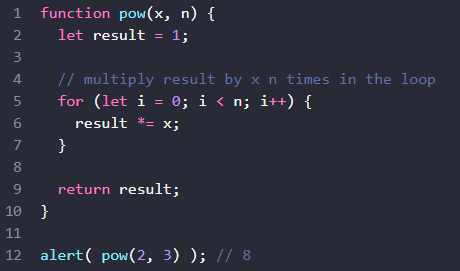# I don't understand this for loop help!I don’t understand what result represent for & what *=x is. Please explain these code.
Tq.

Try adding some `console.log` statements. What is the value of `result` on each loop iteration?

``````function pow(x, n) {
let result = 1;

for (let i = 0; i < n; i++) {
result *= x;
}

return result;
}
console.log(pow(2,3)); #result: 8
``````

Did you try adding `console.log` statements to see what’s happening?

``````function pow(x, n) {
let result = 1;
console.log("start: result = " + result);

for (let i = 0; i < n; i++) {
result *= x;
console.log("loop iteration " + i + ": result = " + result);
}

console.log("final: result = " + result);
return result;
}
console.log(pow(2,3)); // result: 8
``````

yes, how the result come with 2^3? How does it loop?

Have you used a for loop before?

``````function pow(x, n) {
let result = 1;
console.log("start: result = " + result);

for (let i = 0; i < n; i++) {
result *= x;
console.log("loop iteration " + i + ": result = " + result);
}

console.log("final: result = " + result);
return result;
}
console.log(pow(2,3)); // result: 8
``````

ok, so n is using as an index for *=x for loop.

`n` is how many times the loop occurs. `i` is the index

Thank you for your help